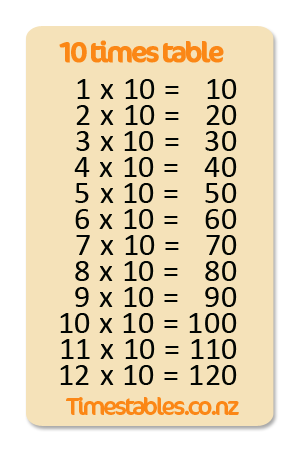# 10 times table

## The 5-step plan

### 10 times table

• 1 x 10 = 10
• 2 x 10 = 20
• 3 x 10 = 30
• 4 x 10 = 40
• 5 x 10 = 50
• 6 x 10 = 60
• 7 x 10 = 70
• 8 x 10 = 80
• 9 x 10 = 90
• 10 x 10 = 100
• 11 x 10 = 110
• 12 x 10 = 120

### Step 1a: View, read aloud and repeat

Step 1a is to get familiar with the table, so view, read aloud and repeat. If you think you remember them it’s time to test your knowledge at step 1b.

### Step 1b: In sequence

Fill in your answers. Once you have entered all the answers, click on ‘Check’ to see whether you have got them all right! If you got all the answers right, practice the 10 times table shuffled in random order.

### Step 3: Shuffled

Practice the 10 times table shuffled. Fill in all answers and press 'check' to see how many you got right.

### Step 4: Multiple choice

Try to answer all the 15 questions right!

### Step 5: Tables Diploma

Answer all the 24 questions right to get the diploma!

### Games

These games give the possibility to repeat the questions and improve the knowledge of the 10 times table. Enjoy the 10 times table games!

## 10 times table memory

Try to find as fast a possible the matching questions and answers!

2
2 x 1
2
2 x 1
2
2 x 1
2
2
2
2
2
2
2
2
2
2

## Welcome to the 10 times table page

This is where you can practise your 10 times table. You can practise the 10 times table in sequence and once you have got the hang of that you can make it a bit harder by practising the sums up in random order. If you want to practise the 10 times table against the clock, you can of course take the speed test. If you want to practise at your leisure, we suggest you print out the 10 times table worksheet and practise with that. What's great about the 10 times table is you just add a 0 to the number to be multiplied and you have the right answer.## Print 10 times table worksheet

Click on the worksheet to view it in a larger format. For the 10 times table worksheet you can choose between three different sorts of exercise. In the first exercise you have to draw a line from the sum to the correct answer. In the second exercise you have to enter the missing number to complete the sum correctly. In the third exercise you have to answer the sums which have been shuffled.

## Description of the 10 times table

This is where you can practice the 10 times table by entering all the answers and then checking how many you got right. There are various ways the tables can be practiced. You are now on the 10 times table page where multiplication calculations can be practiced in sequence. Once you have mastered the table in sequence you can practice the sums in random order. You can learn the tables on a PC, tablet, iPad or mobile phone. You learn this table in year 4 maths.